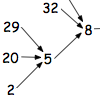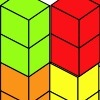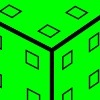#### You may also likeWhat happens when you add the digits of a number then multiply the result by 2 and you keep doing this? You could try for different numbers and different rules.### Triple Cubes

This challenge involves eight three-cube models made from interlocking cubes. Investigate different ways of putting the models together then compare your constructions.### Inky Cube

This cube has ink on each face which leaves marks on paper as it is rolled. Can you work out what is on each face and the route it has taken?

# Journeys in Numberland

##### Age 7 to 11Challenge Level

Although this problem 'just' involved some calculating, explaining the results was a little more tricky.  Charlie, Tom, James and Jonathan from Avenue Junior School looked at the journeys that Tom and Ben made:

Tom starts at B then goes right and gets $5$ more which takes him to $15$.
He then goes up four adding $8$ to his score taking him up to $23$.
He then goes right and gets $5$ more taking him to $28$.
So Tom's score ends up at $28$.

Ben starts at B and goes to the left two squares taking his score to $0$.
He then goes up two squares taking his score to $4$.
He then goes four to the right adding $20$ to his score so he now has $24$.
He then goes up two squares taking his score to $28$.
So Ben's score is $28$, which, oddly enough, is the exact same score as Tom.

Jessica from Egerton Primary School described how her class found lots of different ways of going from B to E on the grid:

We found that in all four of our journeys we got $28$. The whole of the class got $28$ at least two times.  Aidan and I used red and blue crayon to mark which way we went. Aidan and I think that we got $28$ because when you go one way you always have to go back the same way.

Harry, who goes to St Anne's Primary School, had a go at the routes on all three grids.  He wrote:

grid 1) We have found out that they both ended up on $28$.  It doesn't matter how long or short their trial is, it will end up on the same answer.
grid 2) On this grid we have found out that the answer is the same as on the first grid. However, the grid can be as big or as small as you like but you will still have an answer of $28$.
grid 3) On the final grid the answer is always $800,000$.

Harry also played around with the grids a bit, changing addition to multiplication and subtraction to division or vice versa.  This changed the answer but the answer was still always the same, no matter what route you took.

Jack and Skye from Swavesey Primary had a go at the third grid.  They said:

No matter what way you go, you will always end up with the same anwser. We tried a few ways using our computer screen following the journey with our fingers, this was on the multiplication and divide square.
Every time we did it we ended up with the anwser of $800000$.
So we tried even more journeys and always got the same answer of $800000$.

Above, Jessica began to explain why she thought she always got $28$ on the first two grids.  The Maths Galaxy Explorers from North Walsham Junior School clearly thought very hard about this:

We spent our week trying to solve the Journeys in Numberland mathematical challenge. Here is our solution:

Whichever route or sequence you take, you will always get the same answer, as long as the grid is the same. This is because every time there is a times and a divide, or an add and a takeaway, and every time they cancel each other.
For example if you go right for a times by $2$ and left for a divide by $2$, they cancel each other out.
For example: $3 \times 2 = 6$ but if you divide it back ... $6 \div2 = 3$ (which is just halving by the way! and $\times2$ is doubling!) It just cancels out your calculation.
Or $10 + 9 = 19$ OPPOSITE $19 - 9 =10$ tada!!! (It's like dividing and times, it's just undoing again, just like the undo button on your computer!) .

Well done too to Barbara, Cong and Nazra from Arnhem Wharf Primary School who also realised that they would always get the same score for a particular grid.